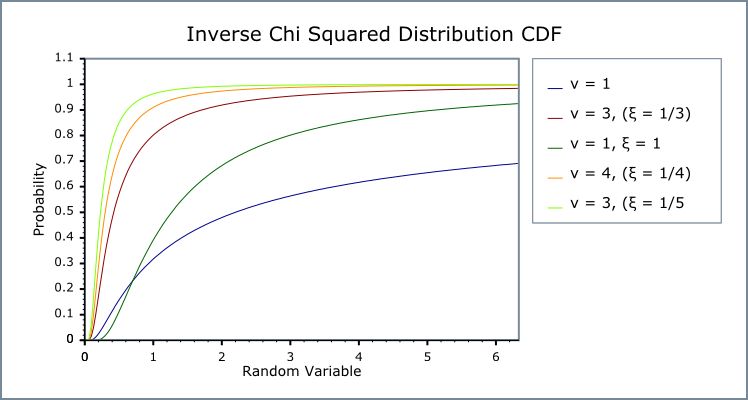#Boost C++ Libraries

...one of the most highly regarded and expertly designed C++ library projects in the world.

This is the documentation for an old version of boost. Click here for the latest Boost documentation.
##### Inverse Chi Squared Distribution

`#include <boost/math/distributions/inverse_chi_squared.hpp>`

```namespace boost{ namespace math{

template <class RealType = double,
class Policy   = policies::policy<> >
class inverse_chi_squared_distribution
{
public:
typedef RealType value_type;
typedef Policy   policy_type;

inverse_chi_squared_distribution(RealType df = 1); // Not explicitly scaled, default 1/df.
inverse_chi_squared_distribution(RealType df, RealType scale = 1/df);  // Scaled.

RealType degrees_of_freedom()const; // Default 1.
RealType scale()const; // Optional scale [xi] (variance), default 1/degrees_of_freedom.
};

}} // namespace boost // namespace math
```

The inverse chi squared distribution is a continuous probability distribution of the reciprocal of a variable distributed according to the chi squared distribution.

The sources below give confusingly different formulae using different symbols for the distribution pdf, but they are all the same, or related by a change of variable, or choice of scale.

Two constructors are available to implement both the scaled and (implicitly) unscaled versions.

The main version has an explicit scale parameter which implements the scaled inverse chi_squared distribution.

A second version has an implicit scale = 1/degrees of freedom and gives the 1st definition in the Wikipedia inverse chi_squared distribution. The 2nd Wikipedia inverse chi_squared distribution definition can be implemented by explicitly specifying a scale = 1.

Both definitions are also available in Wolfram Mathematica and in The R Project for Statistical Computing (geoR) with default scale = 1/degrees of freedom.

See

The inverse_chi_squared distribution is used in Bayesian statistics: the scaled inverse chi-square is conjugate prior for the normal distribution with known mean, model parameter σ² (variance).

The inverse_chi_squared distribution is a special case of a inverse_gamma distribution with ν (degrees_of_freedom) shape (α) and scale (β) where

α= ν /2 and β = ½.

NoteThis distribution does provide the typedef: `typedef inverse_chi_squared_distribution inverse_chi_squared;` If you want a `double` precision inverse_chi_squared distribution you can use `boost::math::inverse_chi_squared_distribution<>` or you can write ```inverse_chi_squared my_invchisqr(2, 3);```

For degrees of freedom parameter ν, the (unscaled) inverse chi_squared distribution is defined by the probability density function (PDF):

f(x;ν) = 2-ν/2 x-ν/2-1 e-1/2x / Γ(ν/2)

and Cumulative Density Function (CDF)

F(x;ν) = Γ(ν/2, 1/2x) / Γ(ν/2)

For degrees of freedom parameter ν and scale parameter ξ, the scaled inverse chi_squared distribution is defined by the probability density function (PDF):

f(x;ν, ξ) = (ξν/2)ν/2 e-νξ/2x x-1-ν/2 / Γ(ν/2)

and Cumulative Density Function (CDF)

F(x;ν, ξ) = Γ(ν/2, νξ/2x) / Γ(ν/2)

The following graphs illustrate how the PDF and CDF of the inverse chi_squared distribution varies for a few values of parameters ν and ξ:##### Member Functions
```inverse_chi_squared_distribution(RealType df = 1); // Implicitly scaled 1/df.
inverse_chi_squared_distribution(RealType df = 1, RealType scale); // Explicitly scaled.
```

Constructs an inverse chi_squared distribution with ν degrees of freedom df, and scale scale with default value 1/df.

Requires that the degrees of freedom ν parameter is greater than zero, otherwise calls domain_error.

```RealType degrees_of_freedom()const;
```

Returns the degrees_of_freedom ν parameter of this distribution.

```RealType scale()const;
```

Returns the scale ξ parameter of this distribution.

##### Non-member Accessors

All the usual non-member accessor functions that are generic to all distributions are supported: Cumulative Distribution Function, Probability Density Function, Quantile, Hazard Function, Cumulative Hazard Function, mean, median, mode, variance, standard deviation, skewness, kurtosis, kurtosis_excess, range and support.

The domain of the random variate is [0,+∞].

NoteUnlike some definitions, this implementation supports a random variate equal to zero as a special case, returning zero for both pdf and cdf.
##### Accuracy

The inverse gamma distribution is implemented in terms of the incomplete gamma functions like the Inverse Gamma Distribution that use gamma_p and gamma_q and their inverses gamma_p_inv and gamma_q_inv: refer to the accuracy data for those functions for more information. But in general, gamma (and thus inverse gamma) results are often accurate to a few epsilon, >14 decimal digits accuracy for 64-bit double. unless iteration is involved, as for the estimation of degrees of freedom.

##### Implementation

In the following table ν is the degrees of freedom parameter and ξ is the scale parameter of the distribution, x is the random variate, p is the probability and q = 1-p its complement. Parameters α for shape and β for scale are used for the inverse gamma function: α = ν/2 and β = ν * ξ/2.

Function

Implementation Notes

pdf

Using the relation: pdf = gamma_p_derivative(α, β/ x, β) / x * x

cdf

Using the relation: p = gamma_q(α, β / x)

cdf complement

Using the relation: q = gamma_p(α, β / x)

quantile

Using the relation: x = β  / gamma_q_inv(α, p)

quantile from the complement

Using the relation: x = α  / gamma_p_inv(α, q)

mode

ν * ξ / (ν + 2)

median

no closed form analytic equation is known, but is evaluated as quantile(0.5)

mean

νξ / (ν - 2) for ν > 2, else a domain_error

variance

2 ν² ξ² / ((ν -2)² (ν -4)) for ν >4, else a domain_error

skewness

4 √2 √(ν-4) /(ν-6) for ν >6, else a domain_error

kurtosis_excess

12 * (5ν - 22) / ((ν - 6) * (ν - 8)) for ν >8, else a domain_error

kurtosis

3 + 12 * (5ν - 22) / ((ν - 6) * (ν-8)) for ν >8, else a domain_error

##### References
1. Bayesian Data Analysis, Andrew Gelman, John B. Carlin, Hal S. Stern, Donald B. Rubin, ISBN-13: 978-1584883883, Chapman & Hall; 2 edition (29 July 2003).
2. Bayesian Computation with R, Jim Albert, ISBN-13: 978-0387922973, Springer; 2nd ed. edition (10 Jun 2009)
 Copyright © 2006-2010 John Maddock, Paul A. Bristow, Hubert Holin, Xiaogang Zhang, Bruno Lalande, Johan Råde, Gautam Sewani, Thijs van den Berg and Benjamin Sobotta Distributed under the Boost Software License, Version 1.0. (See accompanying file LICENSE_1_0.txt or copy at http://www.boost.org/LICENSE_1_0.txt)